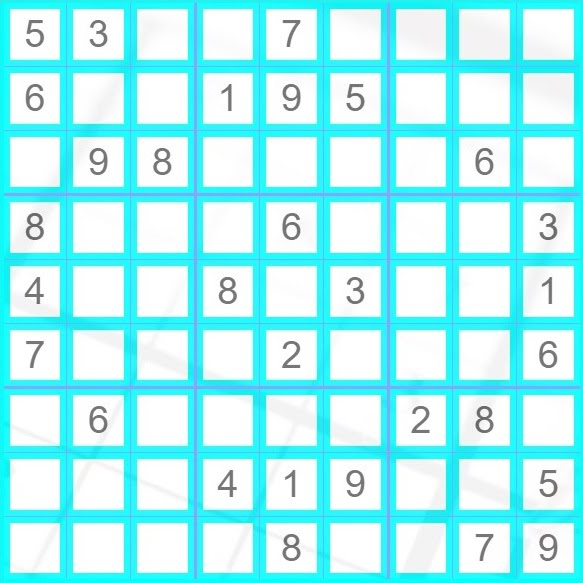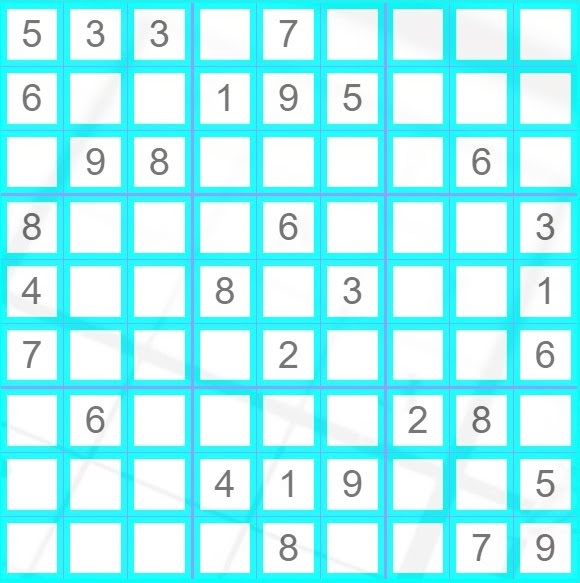# Valid Sudoku

## Introduction

In this article, we will discuss the Valid Sudoku problem. It is an easy question that has been asked in a few competitive coding platforms. Throughout the article, we will discuss the problem statement and some examples and explanations followed by a solution code.

This is a two-part article. This is the second part in which we shall discuss the second variation of the Sudoku Solver problem. The first part of the article can be found here.

Sudoku is a 9x9 puzzle where the board is divided into nine rows and nine columns. It is further divided into nine small grids of 3x3 size each. An element in sudoku is present exclusively in a row or a column, or a grid.

### Problem Statement

Given a 9x9 sudoku board. Determine whether the given sudoku board is valid or not. Only the filled cells need to be validated according to the following constraints:

1. Each row must contain the digits 1-9 without repetition.
2. Each column must contain the digits 1-9 with repetition.
3. Each of the 3x3 sub-boxes of the grid must contain the digits 1-9 without repetition.
• It should be noted that a Sudoku board (filled partially or fully) may or may not be solvable.
• Check only the cells which are filled. Empty cells are to be ignored.
• Each input will be a 2D char matrix. It will contain numbers and dots (.), where a dot represents a blank cell.

### Example and Explanation

Let us see some examples to understand better.

Consider an input sequence-

INPUT_1

{'5','3','.','.','7','.','.','.','.'},

{'6','.','.','1','9','5','.','.','.'},

{'.','9','8','.','.','.','.','6','.'},

{'8','.','.','.','6','.','.','.','3'},

{'4','.','.','8','.','3','.','.','1'},

{'7','.','.','.','2','.','.','.','6'},

{'.','6','.','.','.','.','2','8','.'},

{'.','.','.','4','1','9','.','.','5'},

{'.','.','.','.','8','.','.','7','9'}

The sudoku board will look like this-(source: Sudoku Solver Visualizer)

OUTPUT_1

The Sudoku is Valid.

Let us consider another input sequence-

INPUT_2

{'5','3','3','.','7','.','.','.','.'},

{'6','.','.','1','9','5','.','.','.'},

{'.','9','8','.','.','.','.','6','.'},

{'8','.','.','.','6','.','.','.','3'},

{'4','.','.','8','.','3','.','.','1'},

{'7','.','.','.','2','.','.','.','6'},

{'.','6','.','.','.','.','2','8','.'},

{'.','.','.','4','1','9','.','.','5'},

{'.','.','.','.','8','.','.','7','9'}

This sudoku board will look like-(source: Sudoku Solver Visualizer)

OUTPUT_2

The Sudoku is Invalid.

We are supposed to check whether the given sudoku board is valid or not. A sudoku board is considered valid if it has unique values in each row, column, and grid. In Input_1, all the cells contain unique numbers from 1-9; thus, it is a valid sudoku board. In Input_2, the first row contains element 3 twice (in the same row and same grid), due to which it is invalid sudoku.

## Approach

Let’s head straight to the approach of the Valid Sudoku problem.

• Since we are dealing with sudoku, it is guaranteed that our input will always be a 2D matrix of 9x9 size.
• In the solution code provided, we will be traversing the input matrix row-wise. We will first check if the element is unique in its row, then the column, then the 3x3 grid.
• For this purpose, we will be maintaining two 2D boolean vectors. These will track that at any given position, the elements do not repeat.
• In the solution code, the two vectors are named cols and grid. The two vectors are initialized with false.
• When traversing the sudoku board, three things need to be considered:
• row-wise info: how many rows are to be checked?
• column-wise info: how many columns are to be checked?
• grid-wise info: how many grids are we supposed to check?
• If we think about our approach, we are just looking one row at a time. So for that, a 1D vector is sufficient. For column-wise info, we will be traversing all nine columns, but each column has nine rows. So for that, we make the 2D vector cols. Finally, we will be storing the validation check for all nine 3x3 grids. We can use a map for that, but for simplicity, we will again use a 2D vector of size 9x9.
• Now we start with the main idea. Start loop i from 0 up to 8. For each column i, create a 1D vector row and initialize it with false. Start another loop j from 0 up to 8. For each element in the row at position [i][j] check if it is a digit or not. We do this because we are only concerned with the numbers and not the dot (.) that denotes the blank cell.
• For each digit in a row, we create a variable idx. For our approach, the boolean value at each index of vector row denotes that element is present or not. For example, at ith index, we will store if the digit i is present in that row or not.
• We then perform three checks to check if the element idx is already present in our sudoku or not.
• We first check if idx is already present in that row or not. If present, we return false and exit the function. If not present, we set the idxth element in the row vector as true.
• Then, we check if idx is present in the column. To do so, we check the value at cols[j][idx]. If it is true, we return false and exit our function. Else, we update it to true.
• Finally, we check if idx is present in the grid or not. For that, we find its grid index and store it in the variable gridIdx. To find the grid, we use the formula ((i/3)*3 + (j/3)). If the value at grid[gridInx][idx] is true, we return false and exit the function. Else, we update it to true.
• After both our loops exhaust, we return true, which denotes that our sudoku board is correct and contains unique values at the required positions.

Before moving to the solution code, the readers can write pseudocode to understand the problem better.

The readers can also practice the Valid Sudoku problem on our platform CodeStudio by clicking here: Sudoku?

## C++ implementation of Valid Sudoku

OUTPUT

On running the above code, we get the following output. Since the input board1 is valid sudoku and can be solved further, we display valid. The input board2 is not valid sudoku, as it has repeating numbers in the first row; we display it as not valid.

### Time complexity

In the given approach, we traverse the whole sudoku board once and check each cell. Thus time complexity is,

T(n) = O(n2)

### Space Complexity

In the given approach, we create a 2D boolean vector to store whether a number in a cell is unique or not. Thus,

Space Complexity = O(n2)

1. Is there any alternate method to store the rows, columns, and grids?
Yes, you can store them in other ways. You can create 2D vectors for rows and columns and a map (in C++) to store the grids. For simplification, we have used a 1D vector for rows and 2D vectors for columns and grids.

## Key Takeaways

To summarize the article, we learned about the Valid Sudoku problem. We saw the problem statement, along with some examples and explanations. We discussed our approach thoroughly and saw a solution code for the same. We also discussed the time and space complexities along with some FAQs.

Learn more about Data Structures and Algorithms through this course: Basics of C++ with Data Structures and Algorithms.

Want to ace the coding rounds of big tech companies? Try our Attempt Unlimited Online Mock Test Series to start your preparation.

Happy Coding!